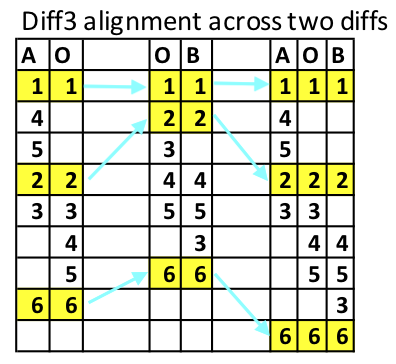## Developing an XML syntax for diff3x

First we look at the trivial but important example of a diff3 for three identical files, i.e. there are no changes. As we are using XML there is a minimum overhead of the start and end tags, so if the example files are all the same, as shown below:

Table 1. Three identical files

A.txtO.txtB.txt
```1
2
3
4
5
6```
```1
2
3
4
5
6```
```1
2
3
4
5
6```

The XML representation would be as shown below and note that white space needs to be preserved, or CDATA could be used.

```<diff3x>1
2
3
4
5
6</diff3x>```

Now we can move on to represent actual changes.

We need an example to show the syntax and we will use the same example as in the previous paper because it also allows us to illustrate how the XML syntax can potentially represent a richer view of the differences. For clarity we repeat the example here. The example is based on this paper, "A Formal Investigation of Diff3" . The example consists of three text files with numbers on each line, the files are denoted A.txt, B.txt and the 'old' file O.txt as shown below:

Table 2. Mismatched sequences

A.txtO.txtB.txt
```1
4
5
2
3
6```
```1
2
3
4
5
6```
```1
2
4
5
3
6```

The way these are combined into the two diffs, A+O and O+B are shown in the table below.The last three columns show how the two diffs are combined. Note that the yellow match shows where all three files align - and this is important because it is the data between these alignment points that are considered as units of change. Now we can look at the diff3 output using the -m option:

```1
4
5
2
<<<<<<< A.txt
3
||||||| O.txt
3
4
5
=======
4
5
3
>>>>>>> B.txt
6```

How might this look as XML? Because we are only looking at a maximum of three files it seems reasonable to have a specific element to represent each one. However, we also need to record the original file names and these could be shown as attributes on the root element. So, the above might be represented in XML as follows.

```<diff3x a="A.txt" b="B.txt" o="O.txt">1
4
5
2<choice3><a>
3</a><o>
3
4
5</o><b>
4
5
3</b></choice3>
6</diff3x>```

The element `<choice3>` introduces a three-way choice between the three original files.